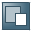Show TOC

###Cost ObjectsYou can plan costs at the material level without reference to an order.

##### Costing at the Material Level Without Reference to an Order

Standard costs for materials are calculated in the application component Product Cost Planning. Costing without reference to an order has the following purposes:

• Calculates the costs for a product before an order to commence manufacturing is placed

• Optimizes the cost of goods manufactured by comparing different cost estimates

• Provides information for the following functions:

• Comparisons between the cost estimate and the order (comparisons between standard costs, planned costs, and actual costs)

• Variance calculation (target/actual comparison)

• Contribution margin accounting

• Inventory valuation

##### Cost Objects

Cost objects are units to which costs can be assigned according to how they were caused using the functions of Cost Object Controlling.

The costs incurred in a company can be assigned to the following cost objects: